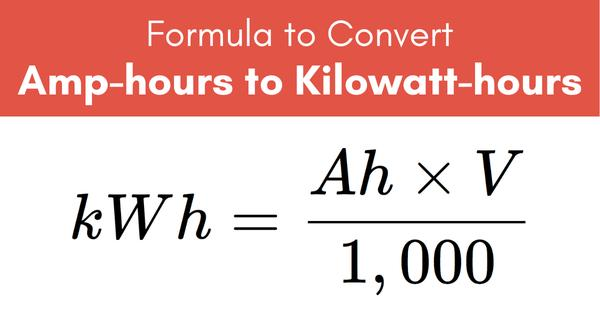Home > HUAWEI > What are kWh and Ah?

# What are kWh and Ah?

A wider hose that lets out a higher volume of water per second (high amperage) but at a lower pressure (low voltage) could make the water wheel spin at the same speed if the total power (wattage) from the combined pressure and current are equal. The total power produced from an electrical circuit (wattage) is determined by the following formula:Power (Watts) = Amps x Volts – so amperage and voltage can vary, but can also result in the same wattage.A kilowatt (kW) is simply a thousand watts. A kilowatt-hour (kWh) – much like an amp hour – is different from a watt. A kilowatt-hour is a measure of energy – how much energy is consumed in a given period. Electric-car batteries are usually measured in kilowatt-hours: you can think of this as being equivalent to the fuel-tank size of a petrol or diesel car. The bigger the battery, the more energy you have and the longer your electric car's range will be.

This is useful for working out charging times, as chargers are always rated in terms of their power, measured in kW. So if you have a 7kW wallbox charger at home, it'll take one hour to deliver 7kWh of electrical energy. Therefore, as a rule of thumb, you can divide a car's battery capacity by the power of a charger to work out the charging time. So a Nissan Leaf with a 40kWh battery that's plugged into a 7kW charger will take around five-and-three-quarter hours to charge (40kWh ÷ 7kW = 5.71 hours).

However, it's important to note that this isn't always true, especially in the case of fast or rapid chargers, which typically use a DC supply. In these cases, charging the final 20% of a nearly full battery will take longer than the first 20% of an empty one, as it becomes increasingly difficult to cram the energy into the cells at such a high rate. This is why you'll see many manufacturers quoting '10-80%' rapid-charge times. And because that final 20% is harder to fill using a rapid charger, many drivers choose to leave public charging stations once they've reached 80% rather than wait longer for the battery to fill up completely.